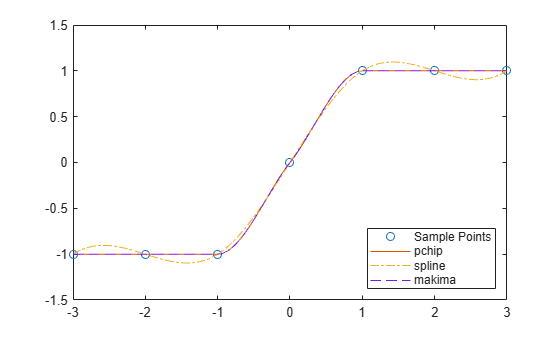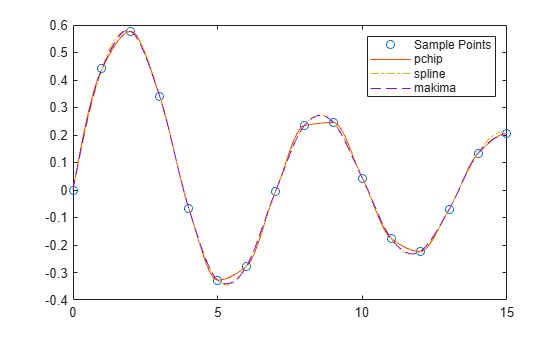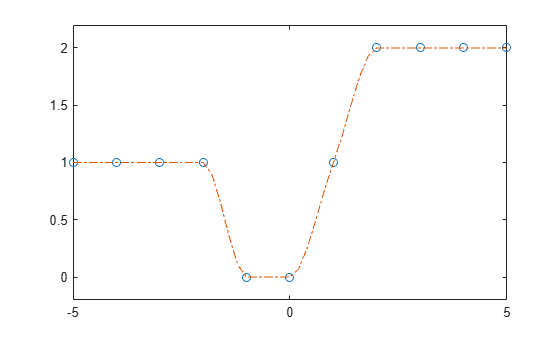# pchip

Piecewise Cubic Hermite Interpolating Polynomial (PCHIP)

## Syntax

``p = pchip(x,y,xq)``
``pp = pchip(x,y)``

## Description

example

````p = pchip(x,y,xq)` returns a vector of interpolated values `p` corresponding to the query points in `xq`. The values of `p` are determined by shape-preserving piecewise cubic interpolation of `x` and `y`.```

example

````pp = pchip(x,y)` returns a piecewise polynomial structure for use with `ppval` and the spline utility `unmkpp`.```

## Examples

collapse all

Compare the interpolation results produced by `spline`, `pchip`, and `makima` for two different data sets. These functions all perform different forms of piecewise cubic Hermite interpolation. Each function differs in how it computes the slopes of the interpolant, leading to different behaviors when the underlying data has flat areas or undulations.

Compare the interpolation results on sample data that connects flat regions. Create vectors of `x` values, function values at those points `y`, and query points `xq`. Compute interpolations at the query points using `spline`, `pchip`, and `makima`. Plot the interpolated function values at the query points for comparison.

```x = -3:3; y = [-1 -1 -1 0 1 1 1]; xq1 = -3:.01:3; p = pchip(x,y,xq1); s = spline(x,y,xq1); m = makima(x,y,xq1); plot(x,y,'o',xq1,p,'-',xq1,s,'-.',xq1,m,'--') legend('Sample Points','pchip','spline','makima','Location','SouthEast')```In this case, `pchip` and `makima` have similar behavior in that they avoid overshoots and can accurately connect the flat regions.

Perform a second comparison using an oscillatory sample function.

```x = 0:15; y = besselj(1,x); xq2 = 0:0.01:15; p = pchip(x,y,xq2); s = spline(x,y,xq2); m = makima(x,y,xq2); plot(x,y,'o',xq2,p,'-',xq2,s,'-.',xq2,m,'--') legend('Sample Points','pchip','spline','makima')```When the underlying function is oscillatory, `spline` and `makima` capture the movement between points better than `pchip`, which is aggressively flattened near local extrema.

Create vectors for the `x` values and function values `y`, and then use `pchip` to construct a piecewise polynomial structure.

```x = -5:5; y = [1 1 1 1 0 0 1 2 2 2 2]; p = pchip(x,y);```

Use the structure with `ppval` to evaluate the interpolation at several query points. Plot the results.

```xq = -5:0.2:5; pp = ppval(p,xq); plot(x,y,'o',xq,pp,'-.') ylim([-0.2 2.2])```## Input Arguments

collapse all

Sample points, specified as a vector. The vector `x` specifies the points at which the data `y` is given. The elements of `x` must be unique.

Data Types: `single` | `double`

Function values at sample points, specified as a numeric vector, matrix, or array. `x` and `y` must have the same length.

If `y` is a matrix or array, then the values in the last dimension, `y(:,...,:,j)`, are taken as the values to match with `x`. In that case, the last dimension of `y` must be the same length as `x`.

Data Types: `single` | `double`

Query points, specified as a scalar, vector, matrix, or array. The points specified in `xq` are the x-coordinates for the interpolated function values `yq` computed by `pchip`.

Data Types: `single` | `double`

## Output Arguments

collapse all

Interpolated values at query points, returned as a scalar, vector, matrix, or array. The size of `p` is related to the sizes of `y` and `xq`:

• If `y` is a vector, then `p` has the same size as `xq`.

• If `y` is an array of size ```Ny = size(y)```, then these conditions apply:

• If `xq` is a scalar or vector, then `size(p)` returns `[Ny(1:end-1) length(xq)]`.

• If `xq` is an array, then `size(p)` returns `[Ny(1:end-1) size(xq)`.

Piecewise polynomial, returned as a structure. Use this structure with the `ppval` function to evaluate the interpolating polynomials at one or more query points. The structure has these fields.

FieldDescription
`form`

`'pp'` for piecewise polynomial

`breaks`

Vector of length `L+1` with strictly increasing elements that represent the start and end of each of` L` intervals

`coefs`

`L`-by-`k` matrix with each row `coefs(i,:)` containing the local coefficients of an order `k` polynomial on the `i`th interval, `[breaks(i),breaks(i+1)]`

`pieces`

Number of pieces, `L`

`order`

Order of the polynomials

`dim`

Dimensionality of target

Since the polynomial coefficients in `coefs` are local coefficients for each interval, you must subtract the lower endpoint of the corresponding knot interval to use the coefficients in a conventional polynomial equation. In other words, for the coefficients `[a,b,c,d]` on the interval `[x1,x2]`, the corresponding polynomial is

`$f\left(x\right)=a{\left(x-{x}_{1}\right)}^{3}+b{\left(x-{x}_{1}\right)}^{2}+c\left(x-{x}_{1}\right)+d\text{\hspace{0.17em}}.$`

collapse all

### Shape-Preserving Piecewise Cubic Interpolation

`pchip` interpolates using a piecewise cubic polynomial $P\left(x\right)$ with these properties:

• On each subinterval ${x}_{k}\le x\le {x}_{k+1}$, the polynomial $P\left(x\right)$ is a cubic Hermite interpolating polynomial for the given data points with specified derivatives (slopes) at the interpolation points.

• $P\left(x\right)$ interpolates y, that is, $P\left({x}_{j}\right)={y}_{j}$, and the first derivative $\frac{dP}{dx}$ is continuous. The second derivative $\frac{{d}^{2}P}{d{x}^{2}}$ is probably not continuous so jumps at the ${x}_{j}$ are possible.

• The cubic interpolant $P\left(x\right)$ is shape preserving. The slopes at the ${x}_{j}$ are chosen in such a way that $P\left(x\right)$ preserves the shape of the data and respects monotonicity. Therefore, on intervals where the data is monotonic, so is $P\left(x\right)$, and at points where the data has a local extremum, so does $P\left(x\right)$.

Note

If y is a matrix, $P\left(x\right)$ satisfies these properties for each row of y.

## Tips

• `spline` constructs $S\left(x\right)$ in almost the same way `pchip` constructs $P\left(x\right)$. However, `spline` chooses the slopes at the ${x}_{j}$ differently, namely to make even ${S}^{″}\left(x\right)$ continuous. This difference has several effects:

• `spline` produces a smoother result, such that ${S}^{″}\left(x\right)$ is continuous.

• `spline` produces a more accurate result if the data consists of values of a smooth function.

• `pchip` has no overshoots and less oscillation if the data is not smooth.

• `pchip` is less expensive to set up.

• The two are equally expensive to evaluate.

 Fritsch, F. N. and R. E. Carlson. "Monotone Piecewise Cubic Interpolation." SIAM Journal on Numerical Analysis. Vol. 17, 1980, pp.238–246.

 Kahaner, David, Cleve Moler, Stephen Nash. Numerical Methods and Software. Upper Saddle River, NJ: Prentice Hall, 1988.